Here's a quick tutorial on using some features of Maple in support of your courses. More details can be had by using Maple's online help functions, and by consulting Maple manuals. This is by no means a comprehensive account of these aspects of Maple, and programming in Maple is not addressed at all.

### Starting and Stopping Maple

There are a number of ways Maple can be invoked. If you are at a workstation, the full graphical front end to Maple can be brought up by invoking Maple from a terminal window by typing xmaple or maple -x at the terminal window's prompt machine_name\$. Bringing Maple up this way uses the Java runtime environment which can be very slow. For a faster, lighter Maple session, bring up graphical Maple environment by typing the command maple -cw at the prompt in the command window. The interface is different, but it retains all the functionality of the Java version.

If you are connecting from a different site (for example, your home PC) to telnet.cs.uleth.ca, or don't need or want the graphical front-end to Maple, you can invoke Maple simply by typing maple at the prompt.

To quit from a graphical session (invoked as xmaple or maple -x or maple -cw), go to the "File" menu and select the option "Exit". Wait a few seconds before logging out, as it will take a few seconds for Maple to clean up after itself before returning the prompt in the terminal window from which you started Maple.

If you are running a text-only session (invoked by maple), simply type quit at the Maple prompt. The command quit is one of the few exceptions to Maple's syntax of requiring either a semicolon or colon following a command. Maple will then exit, print a brief summary of statistics concerning your session, and return your machine's prompt.

### Linear Algebra with Maple

Maple provides some very handy functions for dealing with linear algebra. Below is a Maple session illustrating some of the functionality of the linear algebra tools. Commentary is interspersed with the session. Maple also inserts blank lines to ease readability. These have been removed from the sample session.

```nefarius\$ maple
|\^/|     Maple V Release 5 (University of Lethbridge)
._|\|   |/|_. Copyright (c) 1981-1997 by Waterloo Maple Inc. All rights
\  MAPLE  /  reserved. Maple and Maple V are registered trademarks of
<____ ____>  Waterloo Maple Inc.
|       Type ? for help.
> with(linalg);
Warning, new definition for norm
Warning, new definition for trace
[BlockDiagonal, GramSchmidt, JordanBlock, LUdecomp, QRdecomp, Wronskian,
blockmatrix, charmat, charpoly, cholesky, col, coldim, colspace, colspan,
companion, concat, cond, copyinto, crossprod, curl, definite, delcols,
delrows, det, diag, diverge, dotprod, eigenvals, eigenvalues, eigenvectors,
eigenvects, entermatrix, equal, exponential, extend, ffgausselim,
fibonacci, forwardsub, frobenius, gausselim, gaussjord, geneqns, genmatrix,
innerprod, intbasis, inverse, ismith, issimilar, iszero, jacobian, jordan,
kernel, laplacian, leastsqrs, linsolve, matadd, matrix, minor, minpoly,
mulcol, mulrow, multiply, norm, normalize, nullspace, orthog, permanent,
pivot, potential, randmatrix, randvector, rank, ratform, row, rowdim,
rowspace, rowspan, rref, scalarmul, singularvals, smith, stackmatrix,
submatrix, subvector, sumbasis, swapcol, swaprow, sylvester, toeplitz,
trace, transpose, vandermonde, vecpotent, vectdim, vector, wronskian]
```

If you didn't want the long list of components in the linear algebra package appearing, then instead of typing with(linalg); with its trailing semi-colon, you could just type with(linalg): (i.e., use a trailing colon rather than a semicolon to suppress output).

To get help on any of these functions, type ?function-name at the ">" prompt. No trailing semicolon is required.

```> ?cond
linalg[cond] - condition number of a matrix

Calling Sequence:
cond(A)
cond(A, normname)

Parameters:
A        - a square matrix
normname - (optional) matrix norm, must be one of: 1, 2, 'infinity', or
'frobenius'.

Description:
- The function cond computes the ``standard'' matrix condition number, defined
as norm(A) * norm(inverse(A)). The matrix norm is the default employed by
the linalg[norm] function, namely the infinity norm (maximum row sum).

- More generally, cond(A, normname) computes norm(A, normname) *
norm(inverse(A), normname). This is the same measure, but using the
specified norm instead of the infinity norm.

- The command with(linalg,cond) allows the use of the abbreviated form of this
command.
```

In the newest release of Maple, a separate window opens displaying the help file information for this.

Manipulating matrices is very straightforward in Maple.

```> A:=matrix(3,3,[4,1,0,1,4,1,0,1,4]);
[4    1    0]
[           ]
A := [1    4    1]
[           ]
[0    1    4]
> B := [2,1,3];
B := [2, 1, 3]
> multiply(A,B);
[9, 9, 13]
```

Maple is smart enought to know that the vector B must be interpreted as a column vector in this instance.

```> X0 := [1,1,1];
X0 := [1, 1, 1]
> L:=matrix(3,3,[0,0,0,1,0,0,0,1,0]);
[0    0    0]
[           ]
L := [1    0    0]
[           ]
[0    1    0]
> Dg:=matrix(3,3,[4,0,0,0,4,0,0,0,4]);
[4    0    0]
[           ]
Dg := [0    4    0]
[           ]
[0    0    4]
> U:=matrix(3,3,[0,1,0,0,0,1,0,0,0]);
[0    1    0]
[           ]
U := [0    0    1]
[           ]
[0    0    0]
> evalm(A);
[4    1    0]
[           ]
[1    4    1]
[           ]
[0    1    4]
> evalm(L+Dg+U);
[4    1    0]
[           ]
[1    4    1]
[           ]
[0    1    4]
```

Doing matrix inverses? Notice this:

```> evalm(1/Dg);
[1/4     0      0 ]
[                 ]
[ 0     1/4     0 ]
[                 ]
[ 0      0     1/4]
> evalm(X0);
[1, 1, 1]
> X1 := evalm((1/Dg)*(B - (L+U)*X0));
bytes used=1001056, alloc=720764, time=0.33
X1 := [1/4, -1/4, 1/2]
> X2 := evalm((1/Dg)*(B - (L+U)*X1));
[            13]
X2 := [9/16, 1/16, --]
[            16]
> X3 := evalm((1/Dg)*(B - (L+U)*X2));
[31  -3  47]
X3 := [--, --, --]
[64  32  64]
> X4 := evalm((1/Dg)*(B - (L+U)*X3));
[67   -7   99 ]
X4 := [---, ---, ---]
[128  128  128]
> X5 := evalm((1/Dg)*(B - (L+U)*X4));
[263  -19  391]
X5 := [---, ---, ---]
[512  256  512]
> evalf(263/512);
.5136718750
> evalf(-19/256);
-.07421875000
> evalf(391/512);
.7636718750
```

Maple will perform exact arithmetic. To render a fraction as a decimal (or decimal approximation), the evalf function is used, as illustrated above.

Maple can easily cope with row-reductions of matrices. With the matrix A above, the reduced row-echelon form is obtainable with the rref function:

```> rref(A);
[1    0    0]
[           ]
[0    1    0]
[           ]
[0    0    1]
```

### Differentiation

Routine differentiation can be easily managed in Maple. For example, suppose we have calculated that

yp = e2x((7/5)cos x - (1/5)sin x)

is a solution to the differential equation
y'' - y' + 2y = e2x(cos x - 3sin x),

but want to check our solution. We can easily verify this using Maple's build-in ability to differentiate expressions.

First, let us assign a label f to our expression for yp,

```> f:=exp(2*x)*((7/5)*cos(x)-(1/5)*sin(x));
f := exp(2 x) (7/5 cos(x) - 1/5 sin(x))
```

We need first and second derivatives of f, so use the diff operator to compute them and save the results in df and ddf, respectively:

```> df:=diff(f,x);
df :=

2 exp(2 x) (7/5 cos(x) - 1/5 sin(x)) + exp(2 x) (-7/5 sin(x) - 1/5 cos(x))

> ddf:=diff(df,x);
ddf := 4 exp(2 x) (7/5 cos(x) - 1/5 sin(x))

+ 4 exp(2 x) (-7/5 sin(x) - 1/5 cos(x))

+ exp(2 x) (-7/5 cos(x) + 1/5 sin(x))
```

With the first and second derivatives of yp in hand, we compute the left hand side of our differential equation:

```> ddf-2*df +2*f;
2 exp(2 x) (7/5 cos(x) - 1/5 sin(x)) + 2 exp(2 x) (-7/5 sin(x) - 1/5 cos(x))

+ exp(2 x) (-7/5 cos(x) + 1/5 sin(x))
```

There are some cancellations that can be performed here, but we can get Maple to do that for us with the simplify command:

```> simplify(%);
exp(2 x) (cos(x) - 3 sin(x))

>
```

The percent symbol is Maple's shorthand for the output of the previous command.

Achieving this result validates our answer.

### Plotting Functions

Plotting is best done in the X/Window invocation of Maple (start with maple -x or xmaple or maple -cw).

> plot(x * (x-1) * (x+1), x=-5..5);

A simple graph of this cubic polynomial will appear as output. Notice the syntax of the plot command: the first argument is an expression in an independent variable, followed by the range of the variable. By re-issuing the plot command with different intervals, you can "zoom in" or "zoom out" on the graph. Try the previous command with the intervals x=-1.5..1.5 and x=-10..10 to see the effect for yourself. The command ?plot will provide you with additional information about options to the plot command. Also, if you issue the plot command in a Maple text window session, Maple will fashion a reasonable facsimile of the graph using keyboard characters (an example of this follows).

A helpful timesaver in both the text-only and X/Windows forms of Maple is command recall. By using the "up arrow" key, you can recall a previous command and just edit that instead of re-typing the command. In the X/Windows form, the "up arrow" key will move the cursor up through previous lines in your session.

You can define functions in Maple as well for easy subsequent use. For example,

```> f := x -> (x^2 + 3*x)/(x^4 + x + 1);
```

defines the rational function f(x) = (x2 + 3x)/(x4 + x + 1), and subsequent computations with this rational functional can be done using f(x):

```> f := x -> (x^2 + 3*x)/(x^4 + x + 1);
2
x  + 3 x
f := x -> ----------
4
x  + x + 1

> f(1);
4/3

> evalf(f(1));
1.333333333

> plot(f(x), x=-5..5);

+    AAAA
+  AA   AAA
1+  A      AAA
+ A         AA
+AA           AAAA
+A                AAAAAAA
******-4************--2--+--+--+--+--*--+--+--+--+--2--+--+--+*****4*******
AAAAA           0*
AAA         A+
AA        A+
A       A +
A      -1+
A     AA +
A    A  +
A    A  +
A    A-2+
A  AA  +
A  A   +
A  A   +
AAA   +
AA  -3+
```

In an X/Windows session, the graph would be nicely drawn. You might find it interesting to try plotting functions that have singularities, to see how Maple copes with problem functions.

Maple can also handle functions that are piecewise defined. The appropriate command to use is piecewise, and the syntax is
piecewise( 1st condition, 1st function, 2nd condition, 2nd function, etc., otherwise function).
So, if we wanted a function definition that was sin(x) for x < 1, and then 2*x+1 for 1 <= x < 2, and then 1-x^3, we could code it up as

g:=piecewise(x < 1, sin(x), 1 <= x and x< 2, 2*x+1, 1-x^3);

Try graphing g using the plot command to see for yourself. Try using the plotting option discont=true to rid your graph of artifacts of the plotting process.

For the denominator in our rational function f, you might try to see if it vanishes anywhere. Maple has built-in facilities for root-finding. To search for an exact solution to an equation p(x) = 0, use solve(). If this does not produce a solution in closed form, you can look for a solution numerically with fsolve:

```> solve(x^4 + x + 1 = 0, x);
bytes used=1014064, alloc=851812, time=0.28
4
RootOf(_Z  + _Z + 1)
```

This is Maple's way of stating that it was unable to obtain an exact solution. We can continue by searching for a numerical solution:

```> fsolve(x^4 + x + 1 = 0);
```

In this case, no result is returned, so numerical methods failed to produce a solution to the equation. A slight modification of this problem will show you what to expect to see when solutions do exist:

```> solve(x^4 + x + 1 = 4);
4
RootOf(_Z  + _Z - 3)
> fsolve(x^4 + x + 1 = 4);
-1.452626879, 1.164035140
```

Maple can also plot implicitly defined functions. Issue the with(plots) command to load an enhanced graphics library:

```> with(plots);
[animate, animate3d, animatecurve, changecoords, complexplot, complexplot3d,
conformal, contourplot, contourplot3d, coordplot, coordplot3d,
cylinderplot, densityplot, display, display3d, fieldplot, fieldplot3d,
listcontplot3d, listdensityplot, listplot, listplot3d, loglogplot, logplot,
matrixplot, odeplot, pareto, pointplot, pointplot3d, polarplot,
polygonplot, polygonplot3d, polyhedra_supported, polyhedraplot, replot,
rootlocus, semilogplot, setoptions, setoptions3d, spacecurve,
sparsematrixplot, sphereplot, surfdata, textplot, textplot3d, tubeplot]
```

You should spend some time browsing this rich set of tools. For now, suppose we want a plot of the ellipse x2 + 2(y - 1)2 = 4. The implicitplot function is the appropriate tool to use for this task:

```> implicitplot(x^2+2*(y-1)^2 = 4, x=-4..4, y=-4..4);
```

Maple obligingly supplies a graph. implicitplot can be very helpful when drawing the isoclines for a differential equation.

### Plotting Parametric Curves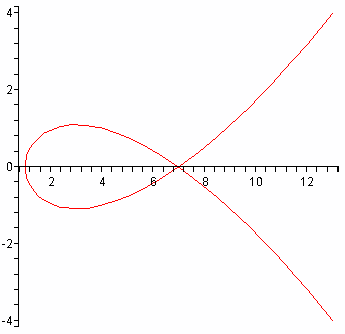Plotting of parametrically defined curves can also be done. For example, to plot the plane curve x = 1 + 3t2, y = t3 -2t, first ensure you have the graphical front end of Maple running (by invoking either xmaple or maple -x or maple -cw from the Unix command line), and then define the functions f and g by the Maple commands

> f:=t->1+3*t^2;
> g:=t->t^3-2*t;

Maple responds by pretty-printing these function definitions. To plot the curves with t ranging from, say, -2 to 2, issue the command

>plot([f(t),g(t),t=-2..2]);

Maple produces the figure at right.

Plotting polar curves produces no great difficulties. To plot a curve expressing r as a function of an angle, first define r as a function of the angle, and then plot the Cartesian equations as above.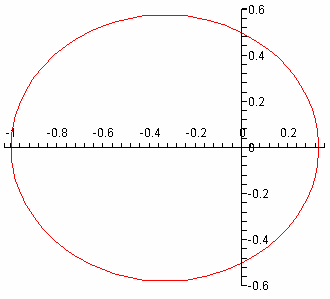For example, to plot the ellipse given by r = 1/(2 + cos(theta)), we define r by r:=theta->1/(2+cos(theta)) to which Maple responds by pretty-printing the function definition, and then issue the plot command

> plot([r(theta)*cos(theta), r(theta)*sin(theta),
> theta=0..2*Pi], scaling=constrained);

Notice that Pi is a built-in constant for Maple. The corresponding figure produced will indeed be that of an ellipse, though if you omit the option scaling=constrained from the plot command, what you'll see will be more like a circle due to Maple's default action of scaling axes to fill out the command window.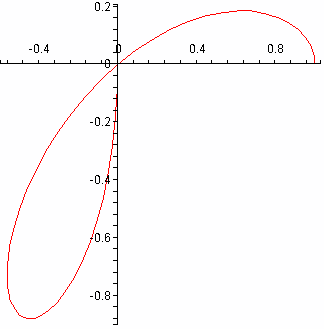A more interesting polar curve is a three-leaved rose, given by r = cos(3*theta). If plotted as for the previous example with, say, r1:=theta->cos(3*theta) used to define r as a function of theta, then the full curve is traced out, of course, with theta varying from 0 to 2*Pi. You can analyse the curve using smaller intervals for theta, though. Using the interval from 0 to Pi will trace out the full curve, and if you restrict theta further, say to [0, Pi/2], using the command plot([r1(theta)*cos(theta), r1(theta)*sin(theta), theta=0..Pi/2]), the figure at the right results.

To plot parametric curves in space requires the use of the plots package, so make sure you have loaded the package into your active Maple session by typing with(plots): into the command window in Maple if you haven't already done so.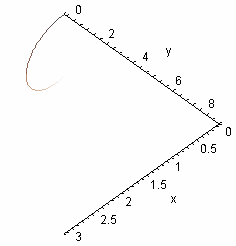Let us plot the parametric space curve x = t, y = t2, z = t3 with t ranging over the interval [0, 3].

We could define functions for each of the coordinates x, y and z as we did for our first parametric curve in the plane, but this one is simple enough to do directly. Issue the command spacecurve([t,t^2,t^3], t=0..3, axes=frame,labels=[x,y,z]); in the Maple command window. Maple produces the figure at the right, which sadly is not very helpful.

The options in the spacecurve command provide axes and labels for the coordinate axes and can be modified or omitted as you see fit.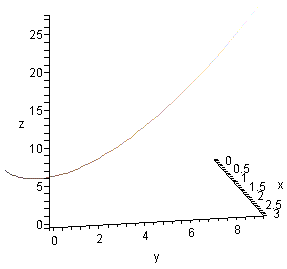Notice that when you move the mouse pointer over the figure, the pointer changes into a curved arrow. If you click and hold the right mouse button while the curved pointer is visible, as the pointer moves the figure itself will rotate. This allows you to view the curve from a number of different points, and gives you a better sense of the three-dimensional character of the plot than would otherwise be the case for a fixed, static, image.

The image at the right is one of the many possible views that result when rotating the image, and the labelling of the coordinate axes is a great help in determining the view you are getting of the curve.

Multiple curves can be plotted on the same graph. To illustrate this, we take as an example the first order PDE used to illustrate the method of characteristics found in the text by Carrier and Pearson: the PDE is

x2ux + uuy = 1
with initial data u = 0 along the line x + y = 1 for x > 0. The curve along which the data are given is a non-characteristic curve, and the characteristics themselves are curves issuing from points along the non-characteristic curve that evolve according to the first order DEs
dx/dt = x2, dy/dt = u, and du/dt = 1,
for which we have
x = s/(1 - st), y = (1/2)t2 + 1 - s, u = t,
where s is used to parametrise the non-characeteristic curve x = s, y = 1 - s, with s > 0.

To plot several of these characteristic curves (starting from the non-characteristic curve at t = 0), we define them first by

x:=(t,s) -> s/(1-s*t);
y:=(t,s) -> t^2/2+1-s;
y:=(t,s) -> t;

which Maple dutifully pretty-prints. The plotting is accomplished by

spacecurve({[x(t,0.1),y(t,0.1),u(t,0.1), t=0..9.6],
[x(t,0.15),y(t,0.15),u(t,0.15), t=0..6.55],
[x(t,0.2),y(t,0.2),u(t,0.2), t=0..4.9],
[x(t,0.3),y(t,0.3),u(t,0.3), t=0..3.25],
[x(t,0.4),y(t,0.4),u(t,0.4), t=0..2.4],
[x(t,0.5),y(t,0.5),u(t,0.5), t=0..1.85],
[x(t,0.6),y(t,0.6),u(t,0.6), t=0..1.55],
[x(t,0.7),y(t,0.7),u(t,0.7), t=0..1.33],
[x(t,0.8),y(t,0.8),u(t,0.8), t=0..1.15],
[x(t,0.9),y(t,0.9),u(t,0.9), t=0..1.02]},
axes=frame,labels=[x,y,u]);

which results in the graph (three views are shown)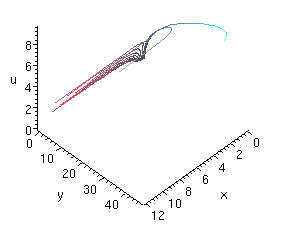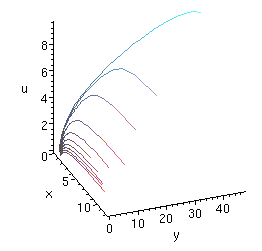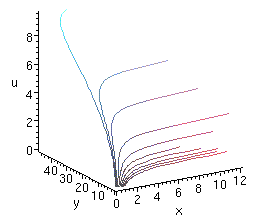### Playing with Surface Plotting

If you haven't yet done so, start up Maple with the graphical front-end by either typing xmaple or maple -x or maple -cw into a command window, and load the graphics library by typing, in the Maple command window, the statement

```> with(plots):
```

We'll explore some possibilities for plotting surfaces in this section. Remember that Maple commands must always be terminated by semicolons, even if the examples shown here don't always reflect this fact.

First, here's how to plot a simple surface given as a function of two variables. We'll use the example of g(x,y) = xy, which has a saddle surface (hyperbolic paraboloid) as its graph. To start, define the function g by issuing the command

```> g:=(x,y)->x*y;
```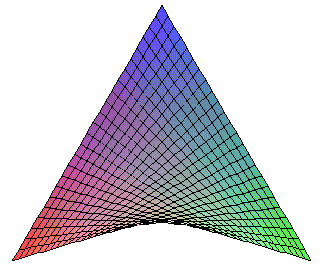Maple will respond by nicely printing your function definition for you. To generate a plot requires the specification of ranges of x and y values. Type

```> plot3d(g(x,y),x=-3..3,y=-3..3);
```

into the command window to generate the graph of g with x and y each ranging over the interval [-3,3]. This will produce the plot seen at the right. If you move your mouse pointer over the graph in the Maple window, the cursor will change into a curved arrow, and if you click and hold the left mouse button and slowly move the curved cursor around, you'll see the plotted image rotate. By playing with the rotations this way, you'll be able to get a sense of how the surface fits into space.

This plot of the saddle surface was done using simple defaults in the plot3d command. To gain some idea of the range of options possible with the plot3d command, type the command ?plot3d,options into the Maple command window. A new window (the help window) will pop open with a description of the plot3d command, along with examples and options. Close the help window and type the command

```> plot3d(g(x,y),x=-5..5,y=-5..5,style=patchnogrid);
```into the command window and Maple will produce a new saddle surface plot, without grid lines. The plot at the right is what you should expect (here, the figure has been rotated to more clearly show the saddle). plot3d has a sizeable number of options, and it is well worth the time to experiment with different options and parameter values.

The plots library of functions can also do plotting of surfaces defined implicitly. For example, to plot the plane with equation 4x - 2y + z = 8, you could issue the command

```> implicitplot3d(4*x - 2*y + z = 8, x=-3..3,y=-3..3,z=-3..3);
```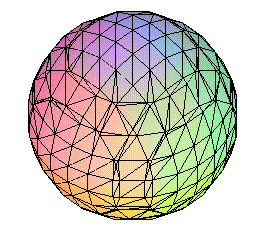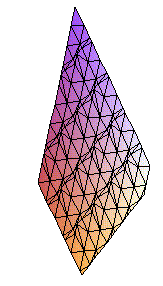and Maple would respond by generating a plot of that portion of the plane found in the rectangular box [-3,3]×[-3,3]×[-3,3]. With a little more sophistication, multiple plots of surfaces can be incorporated into the same graphic. For example, to simultaneously plot a plane and sphere in the same figure, issue the commands

```> plane:=4*x-2*y+z=8;
> sphere:=x^2+y^2+z^2=9;
```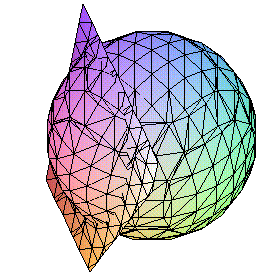This symbolically labels the equations defining each of a plane and a sphere for subsequent, easier reference. If you issue implicitplot3d(plane, x=-3..3, y=-3..3, z=-3..3) and implicitplot3d(sphere, x=-3..3, y=-3..3, z=-3..3) commands, then you will generate separate plots of the plane and the sphere, as illustrated above at right. To incorporate both surfaces in the same plot, though, issue the single command implicitplot3d({plane,sphere}, x=-3..3, y=-3..3, z=-3..3) and Maple will produce the figure at right (this time presented full size from the Maple session used to generate these graphics).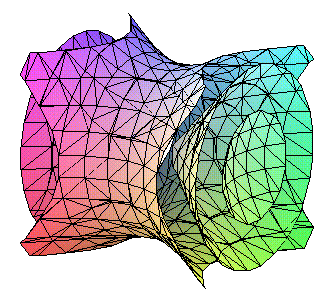Notice that the different surfaces being simultaneously plotted in the one implicitplot3d are enclosed in curly braces {...} and separated by commas. The same idea can be used without resorting to individually labelling each constituent equation. For example, if we wished to plot the three level surfaces f(x,y,z) = -1, f(x,y,z) = 0 and f(x,y,z) = 1 for the function f(x,y,z) = x2 - y2 + z2, then first define the function in the command window by typing f:=(x,y,z)->x^2-y^2+z^2 and then issue the command implicitplot3d({f(x,y,z)=-1, f(x,y,z)=0, f(x,y,z)=1}, x=-1.2..1.2, y=-1.2..1.2, z=-1.2..1.2) to produce the striking figure at the right (or above, depending on your browser and the width you've selected for the window). As before, if you use the mouse to rotate the surfaces, additional insight can be had into how these surfaces are placed in space (in this case, a hyperboloid of one sheet wraps around a cone through the origin which in turn has each nappe containing one component of an hyperboloid of two sheets).

Maple can also plot parametrised surfaces. For example, for the surface with chart (or patch)

x(u,v) = (u cos v, u sin v, u + log cos v)

where v is restricted to the open interval from -Pi/2 to Pi/2, we can get an image of this surface (for u restricted to (0,1) and v restricted to (-1.4, 1.4)) by issuing the plot command
plot3d([u*cos(v),u*sin(v),u+log(cos(v))], u=0..1, v=-1.4..1.4, axes=frame,labels=[x,y,z]) .

The result is shown below.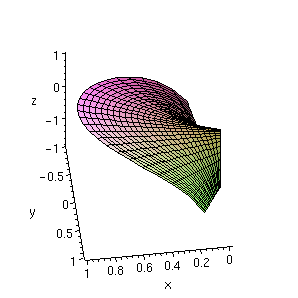Happy plotting!

### Other Plotting

The plots library contains other specialised tools for plotting. Here, we just mention the use of the gradient plotting function gradplot.

If you have not done so, load the plots library into Maple by typing the command with(plots); into the command window. Then try the command

```gradplot(x^3*y^2/(x^2+y^2),x=-2..2,y=-2..2);
```

Maple responds by generating a plot of the gradient vector field associated with the function (in this case, the function is x3y2/(x2 + y2)):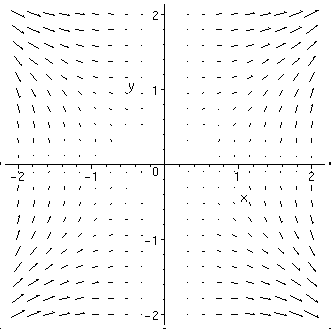Some additional insight into this vector field can be seen by plotting the function and viewing it from several angles. Use the command

```plot3d(x^3*y^2/(x^2+y^2),x=-2..2,y=-2..2,axes=BOXED);
```
and view it from several different angles to try to reconcile the gradient field plot with the surface plot you see.

### Some DE-specific Functions

Maple has a library of functions for use in manipulating differential equations, DEtools. Load this now:

```> with(DEtools);
[DEnormal, DEplot, DEplot3d, DEplot_polygon, DFactor, Dchangevar, GCRD, LCLM,
PDEchangecoords, RiemannPsols, abelsol, adjoint, autonomous, bernoullisol,
buildsol, buildsym, canoni, chinisol, clairautsol, constcoeffsols,
convertAlg, convertsys, dalembertsol, de2diffop, dfieldplot, diffop2de,
eigenring, endomorphism_charpoly, equinv, eta_k, eulersols, exactsol,
expsols, exterior_power, formal_sol, gen_exp, generate_ic, genhomosol,
hamilton_eqs, indicialeq, infgen, integrate_sols, intfactor, kovacicsols,
leftdivision, liesol, line_int, linearsol, matrixDE, matrix_riccati,
moser_reduce, mult, newton_polygon, odeadvisor, odepde, parametricsol,
phaseportrait, poincare, polysols, ratsols, reduceOrder, regular_parts,
regularsp, riccati_system, riccatisol, rightdivision, separablesol,
super_reduce, symgen, symmetric_power, symmetric_product, symtest,
transinv, translate, untranslate, varparam, zoom]
```

The command DEplot is especially useful in the early part of the study of differential equations.

For example, to build a direction field for the DE dy/dx = x2 - y2 over a grid in the x-direction from -4 to 4, and in the y-direction from -3 to 3, enter:

```> de := D(y)(x) = x^2 - y(x)^2;
2       2
de := D(y)(x) = x  - y(x)

> DEplot(de,y(x),x=-4..4, y=-3..3, arrows=THIN,scaling=constrained);
```

The first line of input is the statement of the differential equation in Maple terms, with y's dependence on x explicitly stated. The derivative is expressed as D(y)(x). The entire DE has been labelled as de so that we don't have to re-type in subsequent commands.

The DEplot command is shown with a number of options (use ?DEplot to see more), and with an X/Windows Maple session running, you will obtain an attractive plot of the direction field for this DE.

A slight change to this DEplot command will also graph solutions to initial value problems (IVPs). Instead of the DEplot we used above, issue the command:

> DEplot(de,y(x),x=-4..4, {[y(-2)=0]}, y=-3..3);

Maple will produce a direction field plot again over the same grid in the xy-plane, but now with a curve superimposed on the grid which shows the solution to the IVP with initial data y(-2) = 0.

DEplot can be used with coupled systems of differential equations in much the same fashion. Suppose we want to analyse the system (a competition model)

(d/dt)N1 = N1((1 - N1/5) - N2/10)
(d/dt)N2 = N2((1 - N2/5) - N1/10)

The system can be described by the Maple definition

> de2 := [diff(N1(t),t)=N1*((1-N1/5) - N2/10), diff(N2(t),t)=N2*((1-N2/5) - N1/10)];

To plot the direction field with solution curves issuing, at t = 0, from points (N1, N1) = (2,1), (6,1), (6,6) and (1,7), we would use DEplot in the form

```> DEplot(de2, [N1(t),N2(t)], t=0..5,
>	{[N1(0)=2,N2(0)=1],[N1(0)=6,N2(0)=1],
>	[N1(0)=6,N2(0)=6],[N1(0)=1,N2(0)=7]},
>	N1=0..8, N2=0..8);
```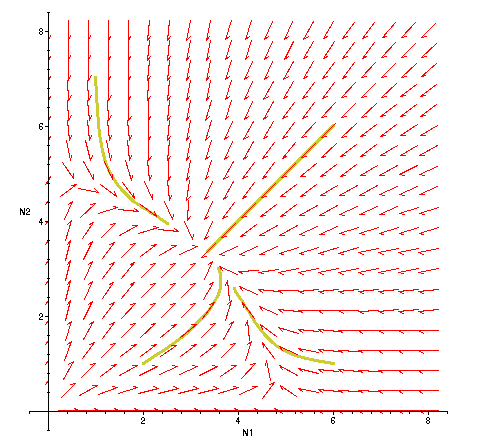Maple then obligingly produces the figure displayed at the right (it will squawk, however, about variable names).

From the illustration produced, we can clearly see the equilibrium point "attracting" solutions issuing from different parts of the N1N2-plane. The equilibrium point, by the way, is readily found to be (10/3, 10/3), and the lines in the first quadrant for which either (d/dt)N1 = 0 or (d/dt)N2 = 0 are easily determined as

N2 = 10 - 2N1

and

N2 = 5 - N1/2,

respectively.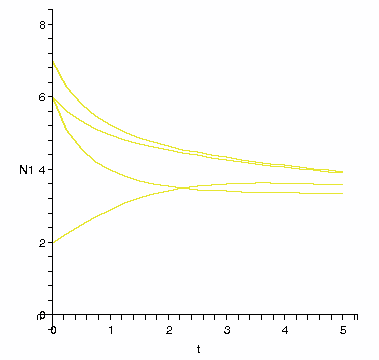If we alter our last DEplot command to

```> DEplot(de2, [N1(t),N2(t)], t=0..5,
> {[N1(0)=2,N2(0)=1],[N1(0)=6,N2(0)=1],
> [N1(0)=6,N2(0)=6],[N1(0)=7,N2(0)=1]},
> N1=0..8, N2=0..8,scene=[t,N1]);
```
(i.e., we add the parameter "scene=[t,N1]" at the end of the command) then instead of producing the direction field plot we had obtained before, we now get plots of N1(t), one for each initial value in the solutions presented in the direction field plot.

Maple can also exactly solve limited classes of DEs (and produce numerical solutions, if desired). For example, to solve the second order DE y'' - y' - 2y = x, you could issue the commands:

```
> eq := (D@@2)(y)(x)-D(y)(x)-2*y(x)=x;

(2)
eq := (D   )(y)(x) - D(y)(x) - 2 y(x) = x

> dsolve(eq, y(x));
bytes used=5215952, alloc=2358864, time=2.53
y(x) = 1/4 - 1/2 x + _C1 exp(2 x) + _C2 exp(-x)
```

The dsolve command is the relevant tool here. In the definition of the DE (which here is labelled "eq"), you can see how to express a second-order derivative. In the solution Maple was able to furnish, you'll notice the expression of two arbitrary constants _C1 and _C2. This is what we would expect to happen in the solution of a second order equation. For dsolve output in solving a first-order equation, we would anticipate the presence of a single arbitrary constant in the general solution.

### Sequences and series

Maple can be a useful tool for exploring properties of sequences and series. To illustrate, let us look at a p-series with p = 2. Define the sequence {1/n2} by

a := n -> n^(-2);

Maple responds by pretty-printing the definition of the sequence. To see the first ten terms of this sequence, use the sequence command seq:

seq(a(n),n=1..10);

Maple will respond by computing the sequence elements an for n ranging from 1 to 10, and pretty-printing the result as fractions.

Plotting is a little more involved. First, we generate a list of the sequence elements we want to plot:

sequencepoints := [seq([n,a(n)], n=1..10)];

Of course, you could call the list of ordered pairs (i, ai) something other than sequencepoints. Maple responds by generating the desired list of ordered pairs. To plot an versus i, issue the command plot:
plot(sequencepoints, 0..11, 0..1, style=point, symbol=circle);

The result is a graph like that below.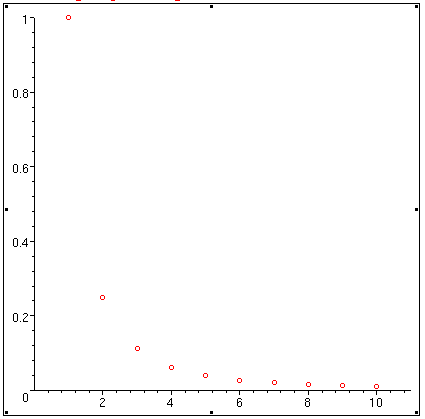If you consult Maple's Help on the plot command, you will see other options available to you. The first range in the plot command, 0..11, indicates the range of the ordinate to be used in the graph. The second range, 0..1, gives the range of the abscissa to be used in building the graph. Try using the same command, this time without specifying the second range. Try the command again, this time specifying the option symbol=cross in the plot command.

Maple can also do limits. We know that 1/n2 goes to 0 as n tends to infinity; so does Maple:

Limit(a(n), n=infinity);

Maple responds by pretty-printing the limit. To get a value, next type

value(%);

The percent sign is Maple shorthand for the output of the previous command. In this case, you should see Maple correctly answer "0" for the value of the limit.

To sum elements of a sequence, type

Sum(a(n), n=1..10);

Maple pretty-prints the sum and to see the value of this partial sum, issue the command

value(%);

Maple responds with the exact value of the sum, expressed as a fraction. To obtain a decimal approximation of this fraction, next issue the command

evalf(%);

This tells Maple to use the machine's floating point arithmetic to compute the fraction, calculator style. Occasionally, Maple is also able to sum an infinite series. For the sum of the full p-series with p = 2, try the command
Sum(a(n), n=1..infinity);

Maple again pretty-prints the series, and to see if Maple can calculate a value for the sum of the series, try

value(%);

In this case, Maple can evaluate the sum of the series, and responds with a pretty-printed exact value. To obtain a decimal approximation to this, again issue the command

evalf(%);

If Maple cannot evaluate a series, it won't and will let you know. Sometimes, Maple can determine if a series diverges, too. For example, define the terms in the harmonic series by

h := n -> 1/n;

As usual, Maple pretty-prints the function definition. Then try summing the harmonic series:

Sum(h(n), n=1..infinity);

Maple pretty-prints the series, but now if you issue the command value(%), Maple responds by printing the infinity symbol to indicate that the series diverges to infinity.

What does Maple do if a series' divergence or convergence can't be determined? Try this exercise: define the sequence of alternating 1s and (-1)s by

d := n -> (-1)^n;

Then trying summing it:

Sum(d(n), n=0..infinity);

As expected, the series is pretty-printed. What results now if you issue the command value(%)?

You can use the Limit command to aid you in applying convergence tests. For example, to apply the ratio test to the series of terms given by

b := n -> 2^n/(2*n)!;

try using the Limit function to compute the limit of the ratio of consecutive terms in the sequence:

Limit(b(n+1)/b(n), n=infinity);

followed by value(%).